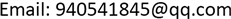1. 引言

2. 滤波器结构2.1. 零序滤波器

2.2. 串联中线滤波器

Z f = X L 1 X C X L 1 + X L 2 + 2 X M + X C

3. 等效电路分析3.1. 零序等效电路

i s n = Z z n Z s n + 3 Z f + Z s + Z z + Z z n i s 0 + 1 Z s n + 3 Z f + Z s + Z z + Z z n u s 0

i z n = Z s n + 3 Z f + Z s + Z z Z s n + 3 Z f + Z s + Z z + Z z n i s 0 − 1 Z s n + 3 Z f + Z s + Z z + Z z n u s 0

Z s n + 3 Z f + Z s + Z z Z s n + 3 Z f + Z s + Z z + Z z n i s 0 ≫ Z z n Z s n + 3 Z f + Z s + Z z + Z z n i s 0

u z n = ( Z s n + 3 Z f + Z s + Z z ) ( Z z n ) Z s n + 3 Z f + Z s + Z z + Z z n i s 0 − Z z n Z s n + 3 Z f + Z s + Z z + Z z n u s 0

( Z s n + 3 Z f + Z s + Z z ) ( Z z n ) Z s n + 3 Z f + Z s + Z z + Z z n ≈ Z z n

u z n = ( Z s n + 3 Z f ) ( Z z n ) Z s n + 3 Z f + Z s + Z z + Z z n i s 0 + Z s n + 3 Z f Z s n + 3 Z f + Z s + Z z + Z z n u s 0

3.2. 三次谐波等效电路

i s n = Z z n Z s n + Z f + Z s + Z z + Z z n i 3 s + 1 Z s n + Z f + Z s + Z z + Z z n u 3 s

4.2. 仿真结果分析

5. 结论